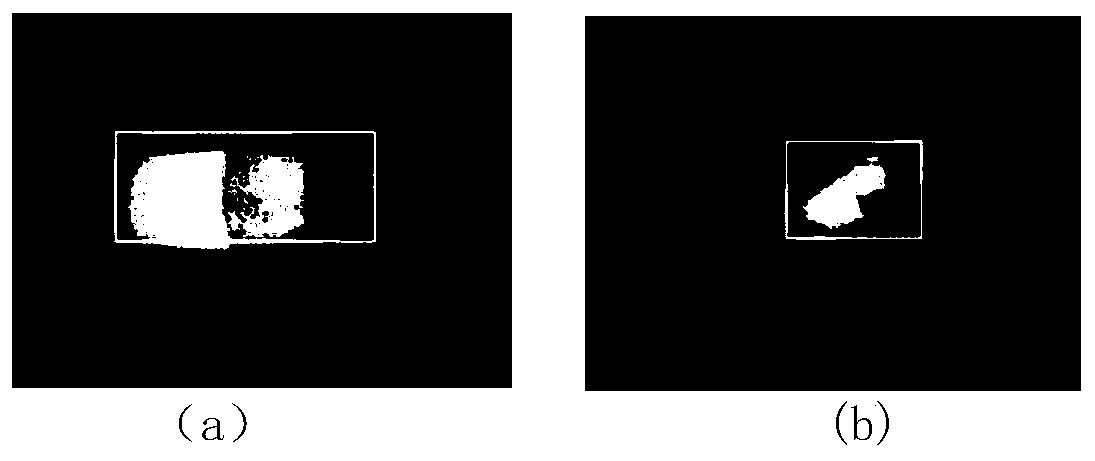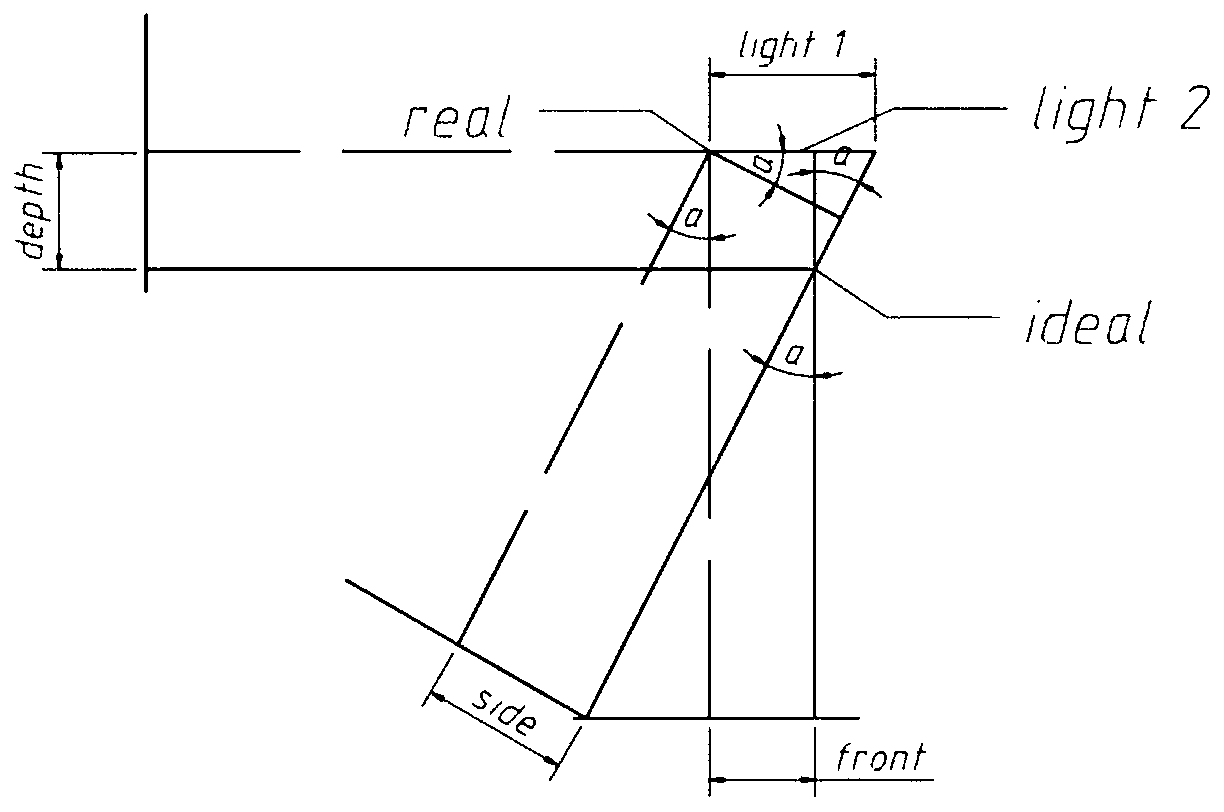# Method for detecting heat source center in three-dimensional space

## A three-dimensional space, center detection technology, applied in the field of computer vision, can solve problems such as large amount of calculation and expensive equipment

Pending Publication Date: 2019-12-24
UNIV OF ELECTRONIC SCI & TECH OF CHINA
6 Cites 0 Cited by

## AI-Extracted Technical Summary

### Problems solved by technology

Generally, laser cameras are used to obtain the depth data of three-dimensional objects, which can obtain accurate surface d...
View more

## Abstract

The invention discloses a method for detecting a heat source center in the three-dimensional space, which belongs to the field of computer vision, and solves the problem of detecting the coordinates of the heat source center in the three-dimensional space. The method comprises the steps of firstly, calibrating an ideal space center as a space coordinate origin; rotating two angles at will; shooting an infrared picture at each of the two positions; then extracting the R channel values of the two infrared pictures to obtain a new picture; cutting the new picture according to the small checks ofa certain size, calculating an R channel average value of each small check, sorting the small checks according to the R values after calculation, calculating the size of a frame of a target heat source according to a heat source proportion condition, at the same time, obtaining the coordinates of the heat source on a two-dimensional image. After the coordinate of the heat source center on a two-dimensional plane is obtained, the depth information can be calculated according to the corresponding geometrical relationship, and finally the coordinate position of the heat source in the three-dimensional space is obtained.

Application Domain

Image enhancementImage analysis

Technology Topic

Seven-dimensional spaceIdeal space +2

## Image

•••## Examples

• Experimental program(1)

### Example Embodiment

 In order to make the objectives, technical solutions, and advantages of the present invention clearer, the specific embodiments of the present invention will be further described below in conjunction with the accompanying drawings, but it is not a limitation to the method of the present invention.
 Attached figure 1 It is the main flow chart of the method of the present invention. The overall scheme is infrared picture preprocessing, target detection of two-dimensional images, and conversion of two-dimensional coordinates to three-dimensional coordinates. The specific steps are as follows:
 (1) Calibrate the ideal space center as the origin of the space coordinates, and rotate it by two angles, take an infrared picture at each of these two positions, and then extract the R channel values ​​of the two infrared pictures to obtain a new picture. Then cut the new picture according to small squares of a certain size, and calculate the average value of the R channel of each small square, and sort the small squares according to the R value after the calculation is completed;
 (2) Calculate the top, bottom, left, and right coordinates of the target heat source according to the proportional relationship of the small square corresponding to the maximum R value, and extract the center coordinate position;
 (3) According to the center coordinates extracted from the infrared images of the two positions, the coordinate information in the three-dimensional space is calculated according to the geometric relationship.
 Step (2) The specific method for extracting the coordinate information of the center is:
 1) From top to bottom, left to right, a preliminary judgment is made on whether each small square is within the range of the target heat source. The judgment method is mainly based on the relationship between the R value of each square and the maximum R value, as shown in the following formula Show:
 R i k*R max
 Where R max Is the square with the highest R channel value, and R i Is the R channel value of the small square that needs to be judged, and k is the scale factor, which needs to be adjusted according to the specific situation;
 2) Judge again the 8 small squares adjacent to each small square that meets the preliminary judgment. If there are n small squares that satisfy step (21), the current square is within the range of the target heat source by default ;
 3) Calculate the upper, lower, left, and right coordinates X of the target heat source according to the position of the small square left , X right ,Z top ,Z bottom And calculate the center coordinates:
 X target =(X left +X right )/2
 Z target =(Z top +Z bottom )/2
 In the above formula, X target Is the horizontal coordinate position, Z target Is the height coordinate position. The test results on the two-dimensional plane are attached figure 2 Shown.
 Step (3) The specific method for calculating the coordinate information in the three-dimensional space according to the geometric relationship is:
 1) According to step 2 to obtain the horizontal and vertical coordinates of the center of the heat source in the horizontal and vertical directions at the two positions, define front and z 1 Is the horizontal deviation and height deviation of the center of the heat source in the front view position, side and z 2 Is the horizontal deviation and height deviation of the center of the heat source in the side view position, depth is the depth distance information of the center of the heat source,;
 2) Taking the ideal space center as the origin of coordinates, define that the side view position is rotated by a certain angle relative to the front view position;
 3) The location of the target heat source is different, and the method of calculating the heat source is also different. It can be divided into six situations, as shown image 3 Shown is a case in which the actual heat source shooting points at the two positions are both left deviations. The calculation method is as follows:
 x=front
 z=(z 1 +z 2 )/2
 light1=side/cosα
 light2=light1-front
 y=depth=light2/tanα
 4) The actual heat source is left deviation at the two shooting points. There is another situation, as attached Figure 4 As shown, the calculation method is as follows:
 x=front
 z=(z 1 +z 2 )/2
 light1=side/cosα
 light2=front-light1
 y=depth=light2/tanα
 5) The actual heat source is offset to the right at the two shooting points. There are also two other cases, one of which is as attached Figure 5 As shown, the calculation method is as follows:
 x=front
 z=(z 1 +z 2 )/2
 light1=side/cosα
 light2=light1-front
 y=depth=light2/tanα
 6) Another situation where the actual heat source at the two shooting points is right deviation is shown in Appendix 6. The calculation method is as follows:
 x=front
 z=(z 1 +z 2 )/2
 light2=side/cosα
 light1=front-light2
 y=depth=light1/tanα
 7) The actual center deviation of the heat source is on the right side of the front-view camera and the left side of the side-view camera, as attached Figure 7 As shown, the calculation method is as follows:
 x=front
 z=(z 1 +z 2 )/2
 light1=side/cosα
 light2=light1+front
 y=depth=light2/tanα
 8) The actual center deviation of the heat source is on the left side of the front-view camera and the right side of the side-view camera, as attached Figure 8 As shown, the calculation method is as follows:
 x=front
 z=(z 1 +z 2 )/2
 light1=side/sinα
 light2=front/tanα
 y=depth=light1+light2
 The above 6 situations include all actual situations, that is, the deviation of the three-dimensional space of the heat source center can be obtained.

## PUM## Description & Claims & Application Information

We can also present the details of the Description, Claims and Application information to help users get a comprehensive understanding of the technical details of the patent, such as background art, summary of invention, brief description of drawings, description of embodiments, and other original content. On the other hand, users can also determine the specific scope of protection of the technology through the list of claims; as well as understand the changes in the life cycle of the technology with the presentation of the patent timeline. Login to view more.
Who we serve
• R&D Engineer
• R&D Manager
• IP Professional
Why Eureka